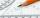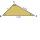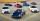Angles ratio

In a triangle ABC true relationship c is less than b and b is less than a. Internal angles of the triangle are in the ratio 5:4:9. The size of the internal angle beta is:

Result

β =  50 °

Solution:Leave us a comment of example and its solution (i.e. if it is still somewhat unclear...):Be the first to comment!To solve this example are needed these knowledge from mathematics:

Do you have a linear equation or system of equations and looking for its solution? Or do you have quadratic equation? See also our trigonometric triangle calculator.

Next similar examples:

1. Smallest internal angleCalculate what size has the smallest internal angle of the triangle if values of angles α:β:γ = 3:4:8
2. Triangle anglesThe angles α, β, γ in triangle ABC are in the ratio 6:2:6. Calculate size of angles.
3. AnglesIn the triangle ABC, the ratio of angles is: a:b = 4: 5. The angle c is 36°. How big are the angles a, b?
4. Find the 9Find the missing angle in the triangle and then name triangle. Angles are: 95, 2x+15, x+3
5. Type of triangleHow do I find the triangle type if the angle ratio is 2:3:7 ?
6. MedianThe median of the triangle LMN is away from vertex N 84 cm. Calculate the length of the median, which start at N.
7. DonutsFind how many donuts each student will receive if you share 126 donuts in a ratio of 1:5:8
8. SavingAllen save € 2860 to buy a car. Yet it lacks save 55% of the car price. How much is the car?
9. 1.5 divided1.5 divided by 1 = w divided by 4
10. Center traverseIt is true that the middle traverse bisects the triangle?
11. Obtuse angleWhich obtuse angle is creating clocks at 17:00?
12. Liters od milkThe cylinder-shaped container contains 80 liters of milk. Milk level is 45 cm. How much milk will in the container, if level raise to height 72 cm?
13. Fifth of the numberThe fifth of the number is by 24 less than that number. What is the number?
14. Unknown numberIdentify unknown number which 1/5 is 40 greater than one tenth of that number.
15. Simple equationSolve for x: 3(x + 2) = x - 18
16. CandyPeter had a sachet of candy. He wanted to share with his friends. If he gave them 30 candies, he would have 62 candies. If he gave them 40 candies, he would miss 8 candies. How many friends did Peter have?
17. EquationSolve the equation: 1/2-2/8 = 1/10; Write the result as a decimal number.